• In degrees, once around a circle is 360°

• In radians, once around a circle is 2π

• A radian measures a distance around an arc equal to the length of the arc's radius

•# Linear vs. Angular Displacement••••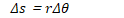# Linear vs. Angular Velocity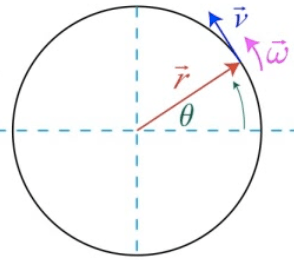••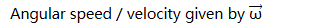•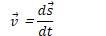•# Direction of Angular Velocity# Converting Linear to Angular Velocity# Linear vs. Angular Acceleration•••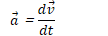•# Centripetal Acceleration# Reference Frames

• A reference frame describes the motion of an observer

• Most common reference frame is Earth
• Laws of physics we study in this course assume we're in an inertial, non-accelerating reference frame

• There is no way to distinguish between motion at rest and motion at a constant velocity in an inertial reference frame

# Calculating Relative Velocities

• Consider two objects, A and B.

• Calculating the velocity of A with respect of reference frame B (and vice versa) is straightforward

• Example:

• Speed of car with respect to the ground

• Walking on a train, speed of a person with respect to the train

•# Linear vs. Angular